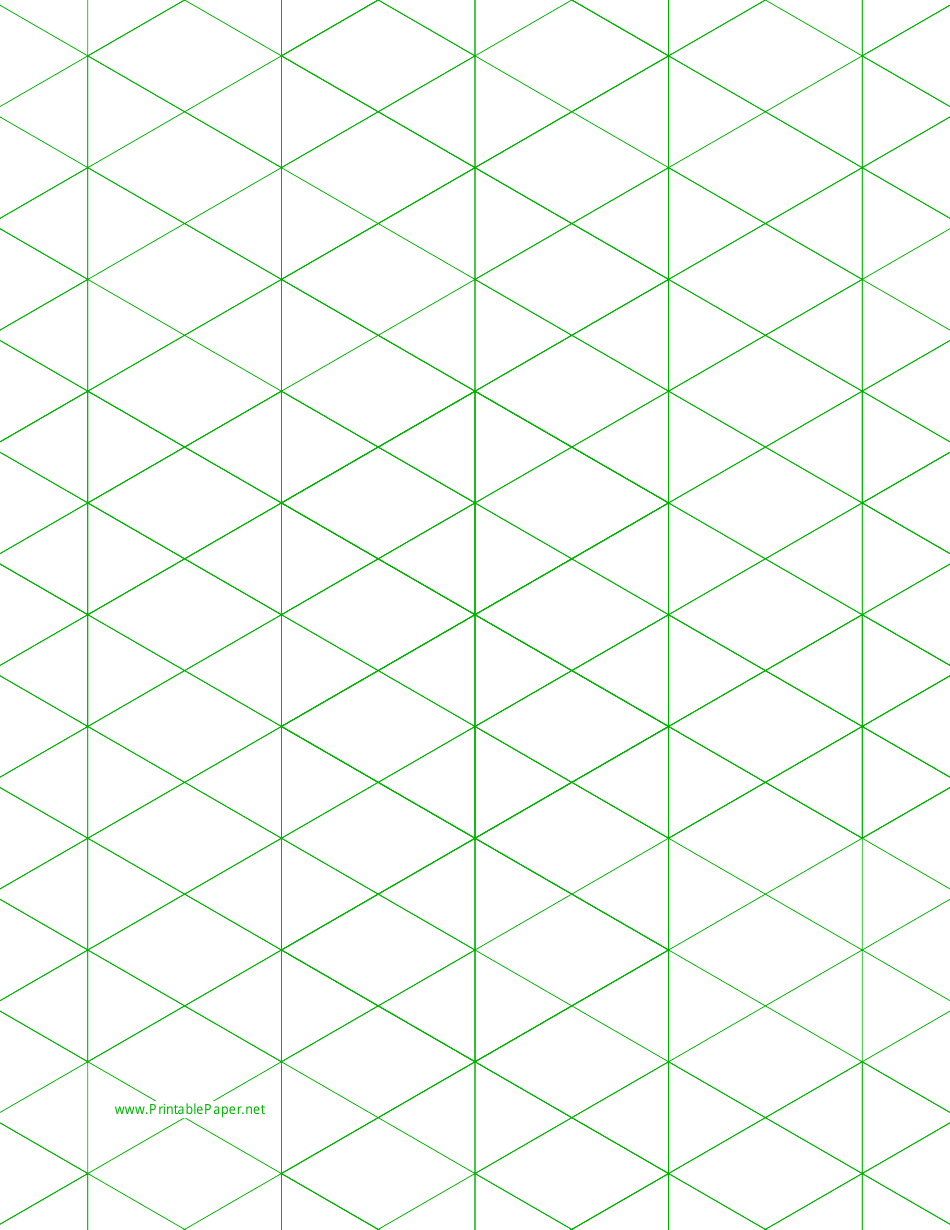# Large Green Half-Square Triangle Paper

Large Green Half-Square Triangle Paper is a type of paper used by quilters for making half-square triangle quilt blocks. It helps in accurately cutting and piecing together fabric pieces to create the desired design.

## FAQ

Q: What is large green half-square triangle paper?
A: It is paper designed specifically for making half-square triangles.

Q: What are half-square triangles?
A: They are quilt squares made by cutting a square in half diagonally to create two triangles.

Q: What is the size of the large green half-square triangle paper?
A: The size may vary, but it's usually larger than regular square paper.

Q: Why is the paper green?
A: The color green is often used in quilting tools and materials for visibility and contrast.

Q: Can I use regular paper instead of the green one?
A: It is better to use the specific paper designed for half-square triangles for accurate results.

•